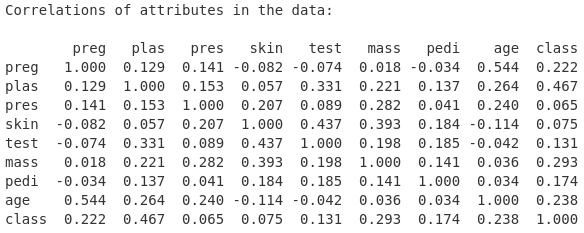# Descriptive Statistics using Pandas: An Introductory Tutorial

In this tutorial, we will learn how to compute descriptive statistics using Python’s Pandas library. We use a well-known dataset in this tutorial. This dataset consists of several medical predictor (independent) variables and one target (dependent) variable, Outcome. Independent variables include the number of pregnancies the patient has had, their BMI, insulin level, age, and so on.

The columns of this dataset are as follows:

1. Pregnancies — Number of times pregnant
2. GlucosePlasma — glucose concentration 2 hours in an oral glucose tolerance test
3. Blood Pressure — Diastolic blood pressure (mm Hg)
4. SkinThickness — Triceps skin-fold thickness (mm)
5. Insulin — Two hours of serum insulin (mu U/ml)
6. BMI — Body mass index (weight in kg/(height in m)²)
7. Diabetes Pedigree Function — Diabetes pedigree function
8. Age — Age in years
9. Outcome — Class variable (0 or 1)

The first eight columns represent the independent variables, and the last column denotes the binary dependent variable. There are a total of 768 entries in the dataset. The outcome variable is set to 1 for 268 entries, and the rest are set to 0.

At first, we import the required package. Here, we use the Pandas read_csv method to read the input CSV file.

`from pandas import read_csv`

We need to specify the input file name. In the following command, the variable filename is a string variable that denotes the name of the input CSV file.

`filename = ‘pima-indians-diabetes.data.csv’`

We now specify the column name. In the following command, names is a Python list that contains the name of each column.

`names = ['preg','plas','pres','skin','test','mass','pedi','age','class']`

Now, the following command is used to read the input CSV file. The Pandas read_csv method is used here. This takes two parameters, namely the filename and the column names. The input CSV file is read into a variable named as data.

`df = read_csv(filename, names=names)`

We can print the data type of the variable data using Python’s type() function.

`print(type(df))`

The output of the above print statement is mentioned below:

`class ‘pandas.core.frame.DataFrame’`

Therefore, the input CSV file is read as a Pandas DataFrame.

We can determine the number of rows and number of columns of the variable data using the shape attribute of the DataFrame df.

`print(df.shape)`

The output of the above print statement is shown below:

`(768, 9)`

The output is a tuple of two numbers. The first number denotes the number of rows and the second number represents the number of columns. Therefore, the input CSV file contains 768 rows and 9 columns.

We can also use the Pandas head() method to print the first five rows of the DataFrame df.

`df.head()`

In Table1, the output of the command df.head() is shown. It shows the initial five rows of the DataFrame df. The column names are shown as we have set them during the reading of the input CSV file to the DataFrame df. Notice that the row indices are set automatically here The row indices start at zero.

We can also determine the data type of each column. Often, columns are called attributes. We can use the dtypes attribute of the DataFrame df to determine the data types of all the attributes.

`print(“Data type of each attribute:\n{}”.format(df.dtypes))`

The output of the above print statement is shown below:

`Data type of each attribute:preg int64plas int64pres int64skin int64test int64mass float64pedi float64age int64class int64dtype: object`

Descriptive statistics

The describe() function of the Pandas DataFrame lists 8 statistical properties of each attribute. They are:

1. Count,
2. Mean,
3. Standard Deviation,
4. Minimum Value,
5. 25th Percentile,
6. 50th Percentile (Median),
7. 75th Percentile,
8. Maximum Value.

The following code will produce the statistical summary of the DataFrame df.

`from pandas import set_option set_option( ‘display.width’ , 100)set_option( ‘precision’ , 3)description = data.describe()print(“Statistical summary of the data:\n”)print(description)`

The output of the above code segment is shown below in Fig. 1.

Distribution of the class attribute

The data considered here is an example of classification data. We can get an idea of the distribution of the class attribute in Pandas.

The following piece of code will breakdown the input data in df based on the class attribute value.

`class_counts = data.groupby(‘class’).size() print(“Class breakdown of the data:\n”)print(class_counts)`

The output of the code segment is shown below:

`Class breakdown of the data:class0 5001 268dtype: int64`

Therefore, there are a total of 768 entries in the dataset. The class variable is set to 1 for 268 entries, and the rest are set to 0.

Correlation between all pairs of attributes:

We can use the corr() function on the Pandas DataFrame to calculate a correlation matrix. For calculating correlation, Pearson’s Correlation Coefficient is used here. Pearson’s Correlation Coefficient assumes a normal distribution of the attributes involved. A correlation of -1 or 1 shows a full negative or positive correlation respectively. On the other hand, a value of 0 shows no correlation at all.

The following code segment is used the determine the correlation between all pairs of attributes in the DataFrame df.

`correlations = data.corr(method = ‘pearson’) print(“Correlations of attributes in the data:\n”) print(correlations)`

The output of the above code segment is shown in Fig. 2.Fig. 2: Correlation between all pairs of attributes in the DataFrame df

Skew of attribute distributions

The skew of each attribute can be calculated using the skew() function on the Pandas DataFrame.

`skew = data.skew() print(“Skew of attribute distributions in the data:\n”) print(skew)`

The output of the above code segment is shown below:

`Skew of attribute distributions in the data:preg 0.902plas 0.174pres -1.844skin 0.109test 2.272mass -0.429pedi 1.920age 1.130class 0.635dtype: float64`

A positive value represents a right-skewed distribution, and a negative value denotes a left-skewed distribution. Values closer to zero corresponds to a less skewed distribution.

This tutorial was originally published in my blog here.

--

--